### Special Relativity

In the $19^\text{th}$ century, the universe seemed a simpler place. It appeared to run like clockwork according to Newton's laws. All that began to change early in the $20^\text{th}$ century, in a golden age for physics that saw the way we view the universe repeatedly turned inside out.

Among the greatest of these changes was Einstein's theory of special relativity, which this course explores. The "relativity" in the theory's name refers to the relative nature of time, length, energy, and momentum, which Einstein declared to be no longer absolute concepts, but different based on the relative motion of an observer or detector.In this chapter, we will explore the motivation for Einstein's theory, but we will begin by recalling how relative motion is woven into Newtonian mechanics.

# Relative Motion of Observers

We will begin our venture into Einstein's theory of special relativity on the high seas. You are exploring a desolate part of the ocean on a raft, and your friend Optimus is above you on a hot air balloon. Your raft moves with the current, while Optimus's balloon moves with the wind.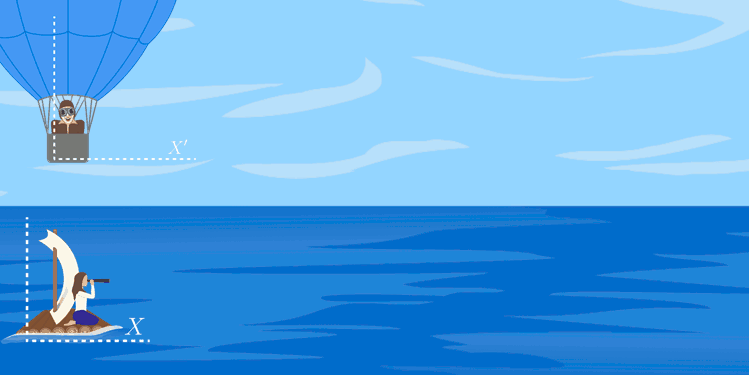While exploring Einstein's theory, we will be concerned about how someone who is making physical measurements—an observer—describes the events and objects around them. You will frequently come across the term reference frame to describe the coordinate system that an observer drags along with them.

Because different observers, such as you and Optimus, can have their own reference frames, the measurements you make of the world will be different.

# Relative Motion of Observers

Optimus is directly above you at $T=0.$ You are both moving East, which you define as the $X$-direction of your coordinate system. He is going faster than you, and his speed is greater than yours by an amount $U.$ This is Optimus's speed relative to you—or more simply, his relative speed.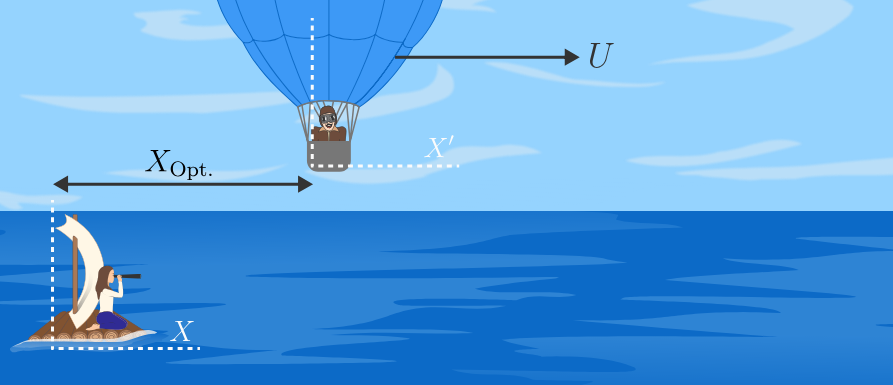Your position in your reference frame is always $X=0.$ What is Optimus's position $X_\text{Opt}$ in your reference frame at time $T?$

# Relative Motion of Observers

You spot a seagull at time $T_\text{gull}$ at a distance of $X_\text{gull}$ ahead of your current position. You call to Optimus and ask him the position of the seagull in his reference frame. He tells you he sees it at $X^\prime_\text{gull}.$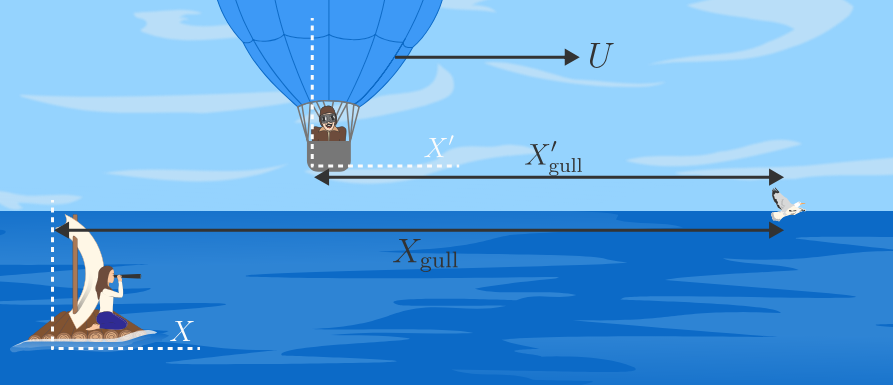Using the answer to the last question, find the relationship between your two measurements. This relationship is called the Galilean transformation and provides a way to convert between your reference frame and Optimus's "primed" reference frame.

Note: at $T=0$ Optimus is right above you, so at this moment, you both are the same distance from the gull.

# Relative Motion of Observers

Although you and Optimus are measuring the same seagull, you report different measurements. This is what is meant by relativity: observers in different reference frames make different (but related) measurements of the same events. In classical mechanics, the Galilean transformation is the tool that translates between spatial positions in different frames.

We will soon see in Einstein's relativity that observers won't agree on spatial positions, or even how much time passes between events, so the transformation between frames will not be so simple. We will need to employ a new tool to relate objects in different reference frames: the spacetime diagram.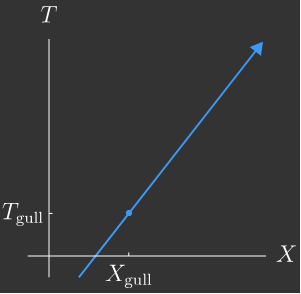Much like position versus time graphs in kinematics, spacetime diagrams depict the set of positions occupied by an object over a range of times. The difference is that on a spacetime diagram, time is on the vertical axis, and a spatial dimension is on the horizontal axis. For example, on a spacetime diagram above, the seagull's motion is plotted in your frame, together with the point when you first observed it.

# Relative Motion of Observers

The trajectories represented on a spacetime diagram are called worldlines. Since observers in different reference frames do not agree on position and speed measurements, they will draw different worldlines on a diagram.

For example, in Optimus's frame, the gull follows the worldline on the diagram below. Relative to you, which is moving faster, Optimus or the gull?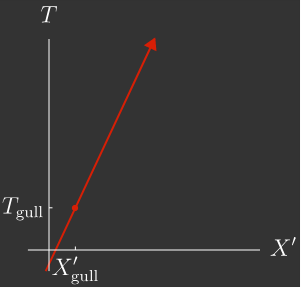Note: in Galilean relativity, observers agree on the time that all events take place, so the time axis of the spacetime diagram in Optimus's frame remains labeled by $T$ instead of $T^\prime.$

# Relative Motion of Observers

Optimus' speed in the air relative to your boat on the water is $U$ and consequently, you and Optimus make different measurements of the gull's speed. Speed along the $X$-axis in your reference frame is $V=dX/dT,$ which we abbreviate as $V=\dot{X}.$ On the other hand, Optimus measures speed $V^\prime=dX^\prime/dT=\dot{X}^\prime$ in his frame.

The Galilean transformation $X^\prime = X- UT$ relates position measurements in your reference frames.You carefully observe the seagull, and in your reference frame, it has velocity $V_\text{gull}.$ Assuming Optimus' relative speed $U$ is constant, what is the seagull's speed $V_\text{gull}^\prime$ in Optimus's primed coordinates?

# Relative Motion of Observers

Suddenly, Optimus pulls a slingshot from his balloon basket. He calls back to you, "I'm low on food, looks like we're having gull tonight!"You know Optimus's slingshot releases with a speed of $V^\prime_\text{shot}$ (in Optimus's reference frame). If you measured the shot's speed in your reference frame, what would you measure?

Recall: $U$ is Optimus's speed relative to your reference frame.

# Relative Motion of Observers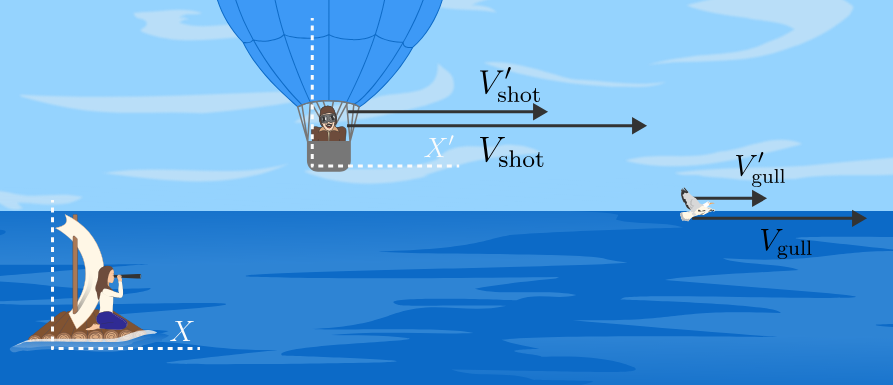The idea of snacking on gull does not whet your appetite, and you hope Optimus misses his shot. In order for it to definitely get away, how should the gull's speed compare to the shot?

Assume gravity is insignificant in this situation.

# Relative Motion of Observers

Because it dovetails with our everyday intuition, Galilean relativity was the tool relating different reference frames until the beginning of the $20^\text{th}$ century, i.e. until a young patent clerk named Einstein demonstrated that the Galilean viewpoint was inconsistent with the properties of light and matter.

Einstein's revisions of relativity were inspired by how observers in different reference frames measure, not gulls or slingshot stones, but the speed of light and high-energy particles.

If Optimus had a rifle with a laser scope, Einstein would have asked, "In a different frame, with what speed does laser light leave the scope?"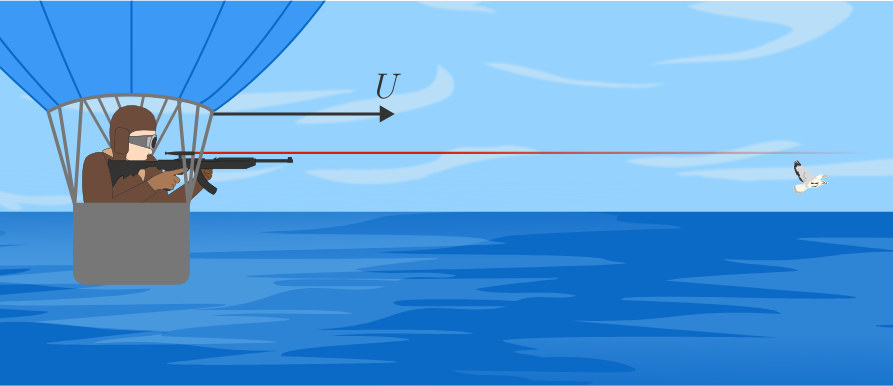This is the central question that Einstein set out to answer when he constructed the theory of relativity. Let's set off in his footsteps to see how he answered this question and why his answer was ultimately correct.

# Relative Motion of Observers

×